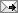A program is a process, not a thing. This also applies to life, the universe, and everything.

## Extending NSBezierPath

Yesterday I wrote about how to extend NSImage so it can save to a file. Today we'll tackle NSBezierPath. NSBezierPath is pretty cool for drawing, but it doesn't support arbitrary regular polygons, just rects and ovals (and lines and arcs). And there's not an easy way to extract the points that make up a path. And if you could extract the points, there isn't a way to draw dots for the points instead of stroking or filling the path. OK, enough already, let's look at some code.

First thing in the code, we'll define some basic trigonometry routines to calculate the points for a polygon. Then we'll create the class itself.

``` from objc import Category from AppKit import NSBezierPath import math def poly_point(center, r, degrees): x = r * math.cos(degrees) + center y = r * math.sin(degrees) + center return x,y def polypoints(center, r, numPoints, degreesRotation=0): if numPoints < 3: raise ValueError, 'Must have at least 3 points in a polygon' rotation = math.radians(degreesRotation) theta = (math.pi * 2) / numPoints return [poly_point(center, r, i*theta+rotation) for i in range(numPoints)] class NSBezierPath(Category(NSBezierPath)): def points(self): points = [] for i in range(self.elementCount()): elem, pts = self.elementAtIndex_associatedPoints_(i) points += pts return points def appendBezierPathWithPolygonWithCenter_radius_numberOfPoints_(self, center, radius, numberOfPoints): ''' Creates a regular polygon ''' pts = polypoints(center, radius, numberOfPoints) self.moveToPoint_(pts) for pt in pts[1:]: self.lineToPoint_(pt) self.closePath() def dot(self): ''' Similar to stroke: and fill:, but draws dots for each point in the path. Dot size is based on linewidth. Not as efficient, because it creates a separate NSBezierPath each time it is called. ''' tmp_path = NSBezierPath.alloc().init() width = self.lineWidth() offset = width / 2 for point in self.points(): rect = (point - offset, point - offset),(width, width) tmp_path.appendBezierPathWithOvalInRect_(rect) tmp_path.fill() OK, hopefully the above is reasonably clear. You can follow along with any calls which are unfamiliar by firing up AppKiDo or the Apple documentation for NSBezierPath. If you're going to use the dot: method a lot you might want to cache the path so you're not creating a new NSBezierPath every time, it depends on what you need. Here's a short script you can run on the command line to create a hexagon and demonstrate fill:, stroke: and dot: from AppKit import NSApplication, NSBezierPath, NSColor, NSImage from Foundation import NSInsetRect, NSMakeRect import image_ext, bezier_path_ext app = NSApplication.sharedApplication() image = NSImage.alloc().initWithSize_((64,64)) image.fillWithColor_(NSColor.clearColor()) image.lockFocus() hex = NSBezierPath.alloc().init() hex.appendBezierPathWithPolygonWithCenter_radius_numberOfPoints_((32,32), 26, 6) NSColor.greenColor().set() hex.fill() hex.setLineWidth_(2) NSColor.blueColor().set() hex.stroke() hex.setLineWidth_(8) NSColor.redColor().set() hex.dot() image.unlockFocus() image.writeToFilePath_('hex.png') Which results in this:Why am I so interested in points and dots? Well, they let me visualize control points for arcs for one thing. Perhaps tomorrow we can explore more along those lines. Posted by: Dethe Elza / Saturday, January 29, 2005```
``` Comments: Post a Comment << Home```
``` ```